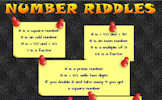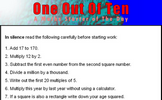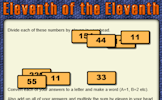# Number sense

### Term 2 starting in week 12 :: Estimated time: 1 weeks

• Round numbers to powers of 10, and 1 significant figure (review)
• Round numbers to a given number of decimal places
• Estimate the answer to a calculation
• Calculate using the order of operations (review)
• Calculate with money
• Covert metric measures of length
• Convert metric units of weight and capacity
• Solve problems involving time and the calendar

For higher-attaining pupils:

• Understand and use error interval notation
• Convert metric units of area
• Convert metric units of volume

This page should remember your ticks from one visit to the next for a period of time. It does this by using Local Storage so the information is saved only on the computer you are working on right now.

## Lesson Starters

Here are some suggestions for whole-class, projectable resources which can be used at the beginnings of each lesson in this block.

### 1st Lesson#### Number Riddles

Can you work out the numbers from the given clues.

### 2nd Lesson#### One out of Ten

This Mathematics lesson starter has been designed for April Fool's Day. Don't be caught out!

### 3rd Lesson#### Peanuts and Buttons

Two questions involving estimating a quantity.

### 4th Lesson#### Maths In School

Find mathematical words which can be made by using the letters in the name of your school?

### 5th Lesson#### Eleventh of the EleventhEnd of block assessments provide a quick progress check at the end of each block of learning to make sure students have understood the content covered. This Scheme of Learning was produced by White Rose Maths and is used here with permission granted on 30th June 2021.